# Graph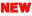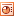Graph Transformations Kinesthetic activity for students. Print out the graphs onto A4 paper, then print the final 6 slides onto accetate and cut up. Pupils use the accetates to show the required graph transformations. Smaller print version, this one prints all the original graphs onto 2 A4 sides, another page on tracing paper for the graphs.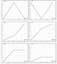Distance-Time Graph Matching Print, cut-out and then match the graphs to the words. Pupils learn to interpret distance-time graphs.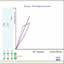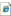Swimming How do the distance-time graphs change as you speed up & slow down the swimmers? Can you make the graphs curve, and how does this affect the swimmers?Skate Boarders How will the graph change as the skate boarders race along the path? What happens as you change thier speeds or stop them completely?Archimedes Learn more about distance-time graphs with Archimedes. What happens to the graph as we fill up the bath? What will if we take out the plug? What will happen to the duck if it overfills?!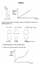Bottles Shapes of graphs. Pupils draw depth/time graphs of differently shaped containers. Please note - this isn't my work, its a great worksheet that I've digitised and reproduced but don't deserve the credit for. Thanks Janice for this.Post Shapes Translations, reflections & rotations make for posting the shapes through the boxesGolf Transformations Can you score under par by cleverly using transformations to get the ball in the hole?!Maths Yoga! Pupils use thier arms to represent the shapes of graphs (2x, x2, x3...) as directed by the teacher (or a pupil) holding up these cards. Once practised with the easier graphs try syncronising it to music, and then try including the harder graphs ((x+2)2, -x3, 4x...) too! Its a good idea to use coloured card to print on as pupils will be able to associate the colour with the shape of the graph. Ie x2 on red, x3 on green etc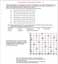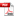Coordinates Great set of exercises on basic coordinates. PDF form.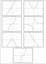Graph Shapes Pupils learn shapes of curved graphs by cutting up and then matching each graph to its equation, sentence & input/output table. Here are the Autograph files used.Some Important Graphs Worksheet with predrawn grids for students to sketch on (nicely!) the graphs of y=x2, y=x3, y=sinx, y=1/x and y=rootx.Graphs, Algebra & Number Machines (and input/output tables & words) Pupils consolidate understanding of straight line graphs by cutting up and then matching each line graph to its equation, sentence, input/output table and number machine.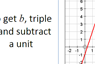Relationships A number machine, algebra, graph, sentence, sequence and input/output table for students to investigate... and hopefully come to the conclusion that all represent the same relationship in different ways. Suggestions for ways to investigate are on slide 4. To extend this rich open task students could create their own set of cards. Lesson plan. A more structured version for less able students. A subsequent activity is for students to complete the number machine, table, sentence and graph from the algebra. Prompt cards for this activity with lesson plan.Label the Straight Line Graphs One page Word file of some straight line graphs fro students to label as y=mx+c.Equation Translations Use your knowledge of translation to move the graph to fit the equations you are givenGraph Shapes Investigation Use Autograph to plot the equations (versions of y=x2) as graphs, then note the differences/similarities to make conclusions.Line Graphs MS Excel program to demonstrate the link between equation, input-output tables & their graphs. This is fantastic for whole class demonstrations including getting pupils to draw on the lines and then using the program to check accuracy.Sketch Graphs Match a sketch graphs to each sentence. Answers included.Transformations helpsheet A few notes on how to describe transformations. Reflection, rotation, enlargement, translation etc.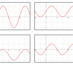Graphs of Related Functions Match-up Activity Graph transformations mathcup activity. Cut out, scramble then match up each equation to its graph.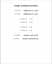Graphs of Related Functions Crib sheet for how functions and their graphs relate to each other. PDF here.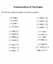Transformations of Trig Graphs Use Autograph to plot these trig graphs alongside the standard trig graphs. Note how the graph transform corresponds to the change in each equation.